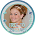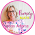Pi Day is just two weeks away.  It's a Monday, so I am planning it now so that we can kick off the week with some fun.  Read on for ideas of what I am planning, but first, some fun Pi Day Facts:

1. Pi is the most recognized irrational number in the world.
2. Comedian John Evans once quipped: “What do you get if you divide the circumference of a jack-o’-lantern by its diameter? Pumpkin π.
3. The symbol for pi (π) has been used regularly in its mathematical sense only for the past 250 years.

1. If the circumference of the earth were calculated using π rounded to only the ninth decimal place, an error of no more than one quarter of an inch in 25,000 miles would result.
2. Egyptologists and followers of mysticism have been fascinated for centuries by the fact that the Great Pyramid at Giza seems to approximate pi. The vertical height of the pyramid has the same relationship to the perimeter of its base as the radius of a circle has to its circumference.
3. The first 144 digits of pi add up to 666 (which many scholars say is “the mark of the Beast”). And 144 = (6+6) x (6+6).
4. Ancient mathematicians tried to compute pi by inscribing polygons with more and more sides that would more closely approach the area of a circle. Archimedes used a 96-sided polygon. Chinese mathematicians Liu Hui inscribed a 192-sided polygon and then a 3,072-sided polygon to calculate pi to 3.14159. Tsu Ch’ung and his son inscribed polygons with as many as 24,576 sides to calculate pi (the result had only an 8-millionth of 1% difference from the now accepted value of pi).

Pi Day is a great day to play with irrational and rational numbers.  First, we talk a little about the definition of irrational numbers and rational numbers.

• Rational numbers are real numbers that can be written as a fraction such as 3, 3/4, 1.57, 3.66666666  or we can think of them as: integers, decimals that repeat or decimals with that terminate (i.e. end).
• Irrational numbers is a real number that can't be written in fraction form.  I think of them as decimals that never repeat.  Some of the more famous irrational numbers include pi, the square root of two and e (Euler's number).  See some examples here (scroll down the page).

Mathsteps has a pretty good diagram of how rational and irrational numbers fit in the big scheme of numbers here.

After a brief discussion, we get down to business "showing" an irrational number of our choice.  I give students three options: beads, paper chains, and cityscapes to represent irrational numbers.

To make the beaded necklaces, students take a variety of beads (you need at least 10 unique beads) and set up a code for each color/shape as a representative of a number.
In the photo above, you can see that there is a round purple bead for 5, an orange bead for 9, etc.

Each student selects an irrational number to make such as pi or root 2. Students string the beads on a string in the order of the numbers of the irrational number.  I let the students decide how many beads (numbers) to put on but give a minimum of 25.  Last year one of the students said upon finishing her necklace: "I'm wearing Pi".  Another student reflected that now she completely understands what it means when a number is an irrational number versus a rational number.  The cityscapes and paper chains are great fun as well and employ similar principals.  Get a full set of the activities here.

For other great ideas for Pi Day, check out the posts from fellow teacher-bloggers below.

Math Mondays is a weekly post sharing tips, ideas, resources, and products for teaching math.  If you have questions or think there is something I should share, you can leave me a message on Facebook or at the store in the question and answer section.

### Share:

#### 3 comments

1.Clearly we love the same Pi Day joke! ;) Neat hands-on activity - thanks for sharing!

2.Love all the quick Pi Day Trivia facts. I like to challenge my students to find a unique fact each year. We also make beads of 100 digits Pi, e, or Root 2! It's a day of math fun.

3.I like the beads activity, that's a great way to make it concrete for kids. Your discussion of rational and irrational numbers put in mind the fact that pi is a transcendental number (not a root). And even though the number line seems crammed full of rational numbers and irrational roots, almost all numbers are transcendental, by virtue of transcendental numbers being uncountable. So cool! Thanks for the post!